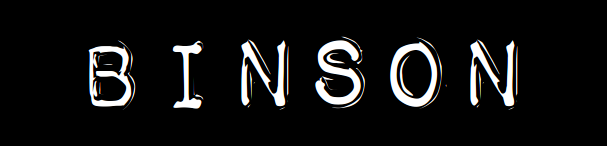Like JSON just faster and simpler

Binson is an exceptionally simple binary data serialization format. It is similar in scope to JSON, but is faster, more compact, and simpler.

Binson has full support for double precision floating point numbers (including NaN, inf). There is a one-to-one mapping between a Binson object and its serialized bytes. This is useful for cryptographic signatures, hash codes and equals operations. And the best feature: Binson has no nulls :-)

Implementations: binson-java, binson-java-light, binson-c, binson-c-light, binson-js, binson-swift, binson-erlang.

Also, checkout BINSON-SCHEMA, an exceptionally light-weight approach to validate the structure of a Binson object. It's like XML Schema for XML, but orders of magnitude less complex.

BINSON-SPEC-1

This is the complete specification of the Binson serialization format, version 1. Refer to it as BINSON-SPEC-1. Written May 2014 by [Frans].

1. Introduction

Binson is a simple, general-purpose data serialization format.

This specification describes the Binson object data structure and how it is serialized to bytes. Like objects of common programming languages, a Binson object has fields. A field is a named and typed value. There are seven value types: five primitive types (boolean, integer, double, string, bytes) and two composite types (array, object). An array is a finite sequence of unnamed, typed values.

2. Format

The bytes of a serialized Binson object follow this [ABNF] syntax.

```object     = begin *field end
field      = string value
value      = boolean / integer / double / string / bytes / array / object
array      = beginArray *value endArray
string     = stringLen utf
bytes      = bytesLen raw
boolean    = true / false

begin      = %x40
end        = %x41
beginArray = %x42
endArray   = %x43
true       = %x44
false      = %x45
double     = %x46 float64
integer    = %x10 int8 / %x11 int16 / %x12 int32 / %x13 int64
stringLen  = %x14 int8 / %x15 int16 / %x16 int32
bytesLen   = %x18 int8 / %x19 int16 / %x1a int32

float64    = 8OCTET ; double precision floation point number [IEEE-754]
int8       = 1OCTET ;  8-bit signed two's complement integer
int16      = 2OCTET ; 16-bit signed two's complement integer
int32      = 4OCTET ; 32-bit signed two's complement integer
int64      = 8OCTET ; 64-bit signed two's complement integer
utf        = *OCTET ; stringLen number of [UTF-8] bytes
raw        = *OCTET ; any sequence of bytesLen bytes
```

3. Rules

A finite sequence of bytes is a serialized Binson object if and only if the following rules are fullfilled.

1. The byte sequence must follow the format of the ABNF rule object.
2. Values must be stored using as few bytes as possible.
3. Fields must be stored in order. The order must be the lexicographical order of the [UTF-8] bytes of the name of the fields.
4. Two fields of the same direct parent object cannot have the same name.
5. Little-endian byte-order must be used.

4. Recommendations

Non-normative recommendations:

1. An object should have less than 100 fields.
2. The size of a serialized Binson object should be less than 40 million bytes.
3. Field names should match the regular expression: [a-zA-Z][a-zA-Z0-9_]{0,49}.
4. Field names should use camel-case and start with a lower-case letter. Acronyms should be treated as words. Examples: g8, httpHeader, customerId.
5. It is recommended that a map (associate array) is stored as a single array. The order of the array values should be: key of first key-value pair, value of first key-value pair, key of second key-value pair, value of second key-value pair and so on.

The reasons for the recommendations are: 1. for readability and feasability of linear search implementations, 2. for feasability of in-memory processing, 3. for readability and inter-operability with other object representations, 4. for consistency, 5. for consistency.

5. References

Page version: 171023.